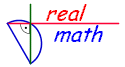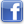Visualize - Practice - Understand successful learning at realmath.de
 Here are interactive dynamic worksheets according to Math Standards. Choose a section! ⇨Numbers   ⇨Algebra   ⇨Geometry   ⇨Measurement   ⇨Data Analysis and Probability

Math overview - Summery of interactive dynamic worksheetsYou like realmath.de

 realmath.de facebookrealmath.de twitter